Most Affordable JEE | NEET | 8,9,10 Preparation by Kota's Top IITian Doctor Faculties

# NCERT Solutions for Class 9 Maths chapter 10 Exercise 10.5 - Circles - Free PDF Download

Class 9Hey, are you a class 9 Student and Looking for Ways to Download NCERT Solutions for Class 9 Maths chapter 10 Exercise 10.5? If Yes then you are at the right place.

Here we have listed Class 9 maths chapter 10 exercise 10.5 solutions in PDF that is prepared by Kota’s top IITian’s Faculties by keeping Simplicity in mind.

If you want to score high in your class 9 Maths Exam then it is very important for you to have a good knowledge of all the important topics, so to learn and practice those topics you can use eSaral NCERT Solutions.

So, without wasting more time Let’s start.

### Download The PDF of NCERT Solutions for Class 9 Maths chapter 10 Exercise 10.5 "Circles"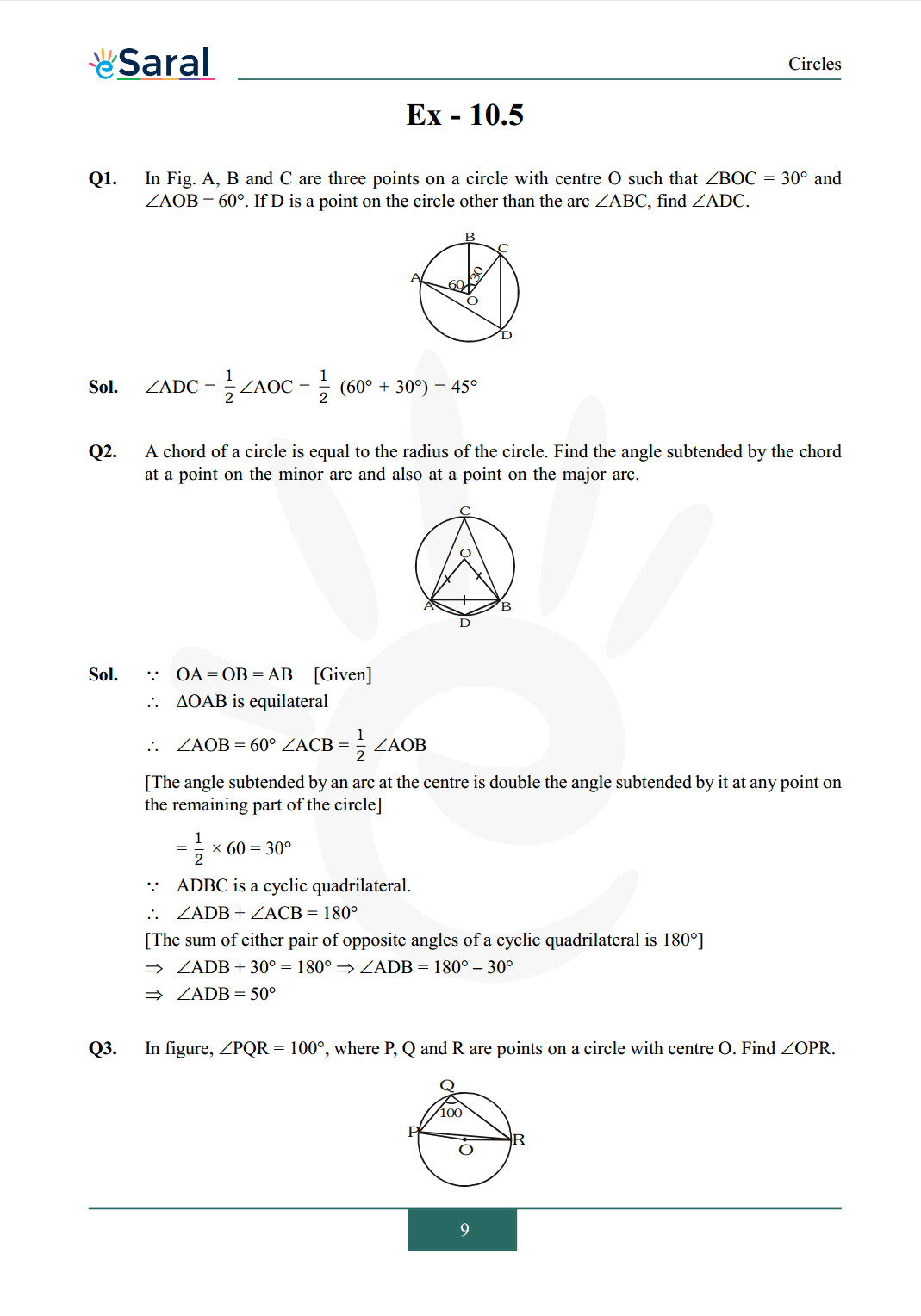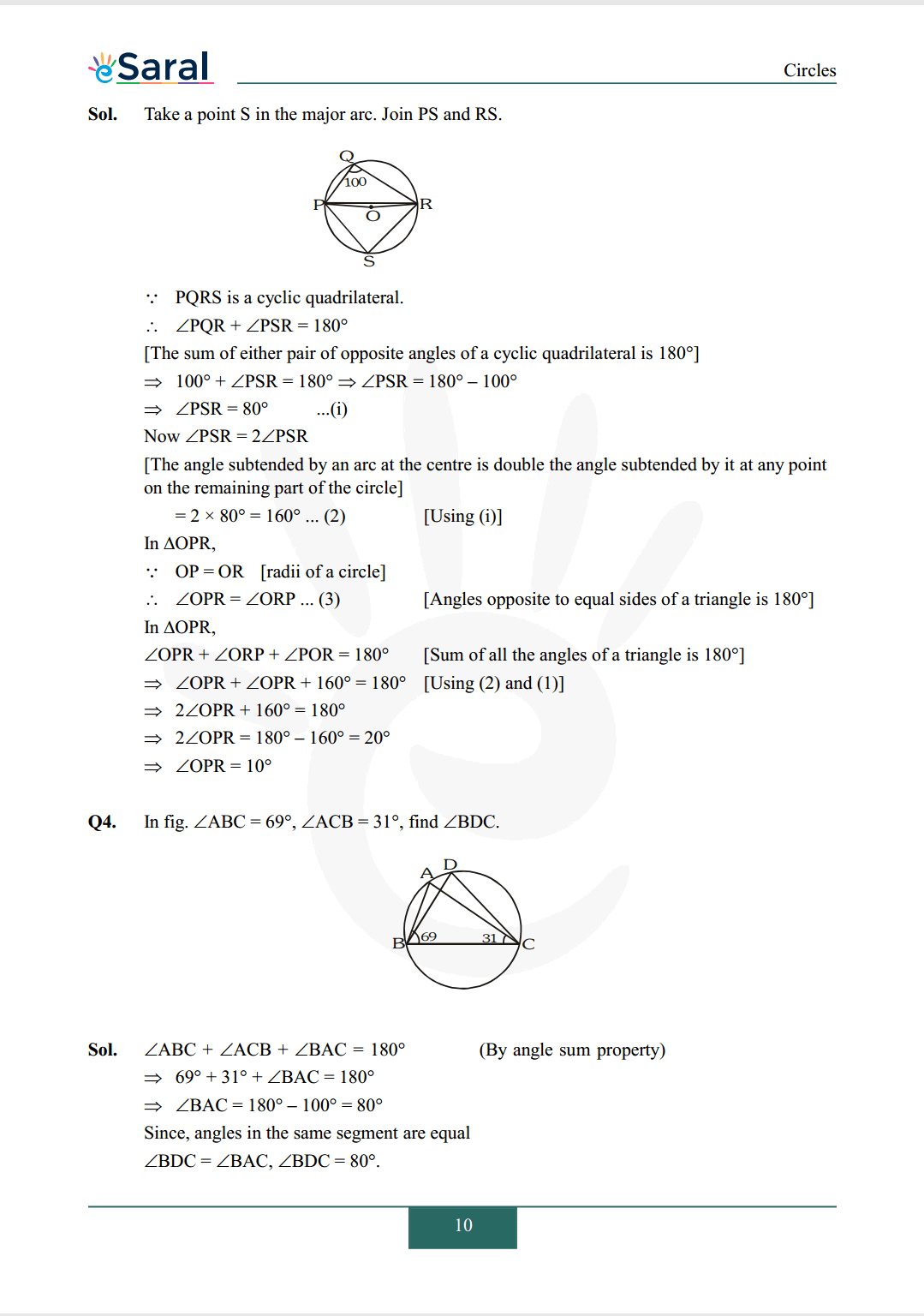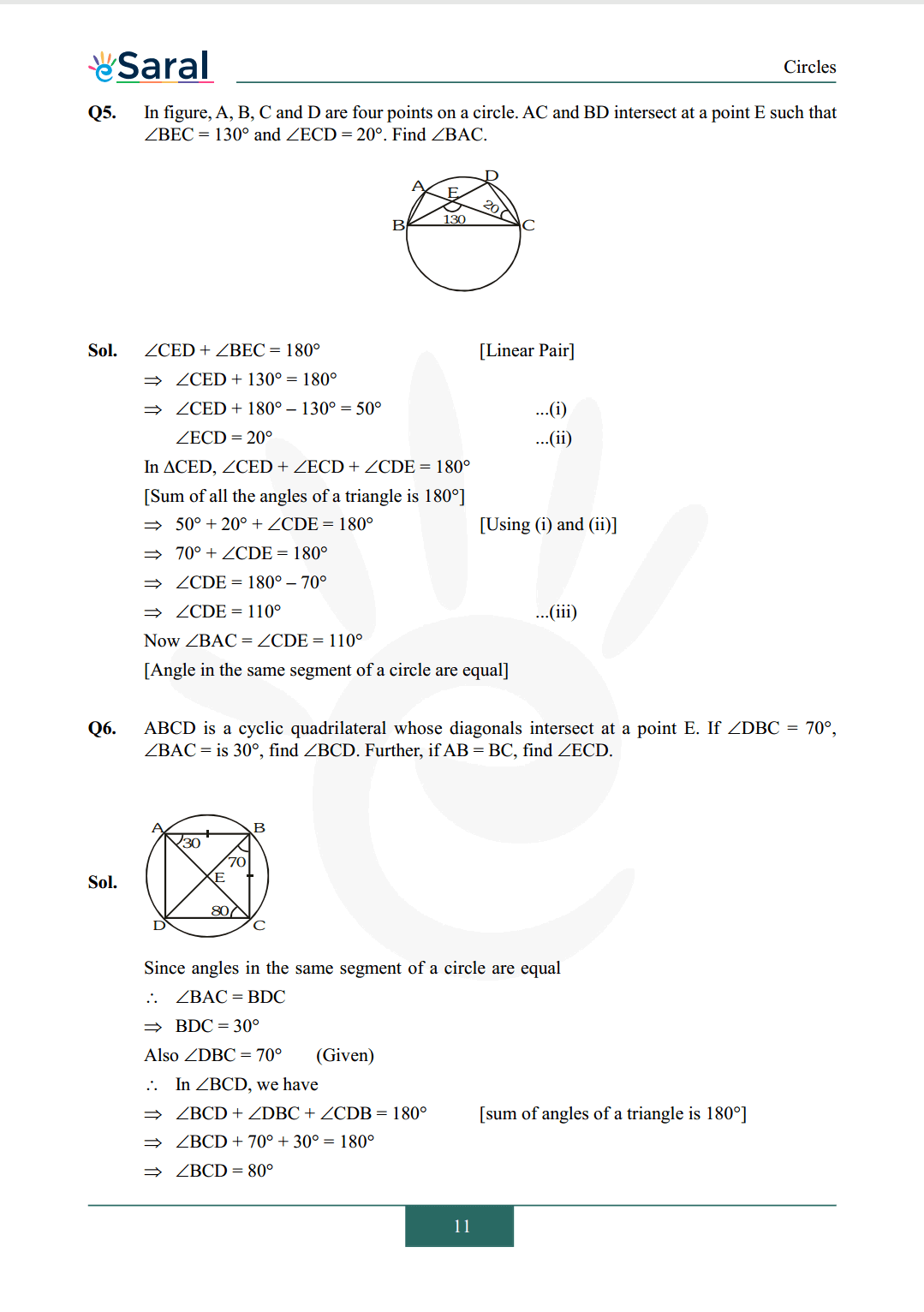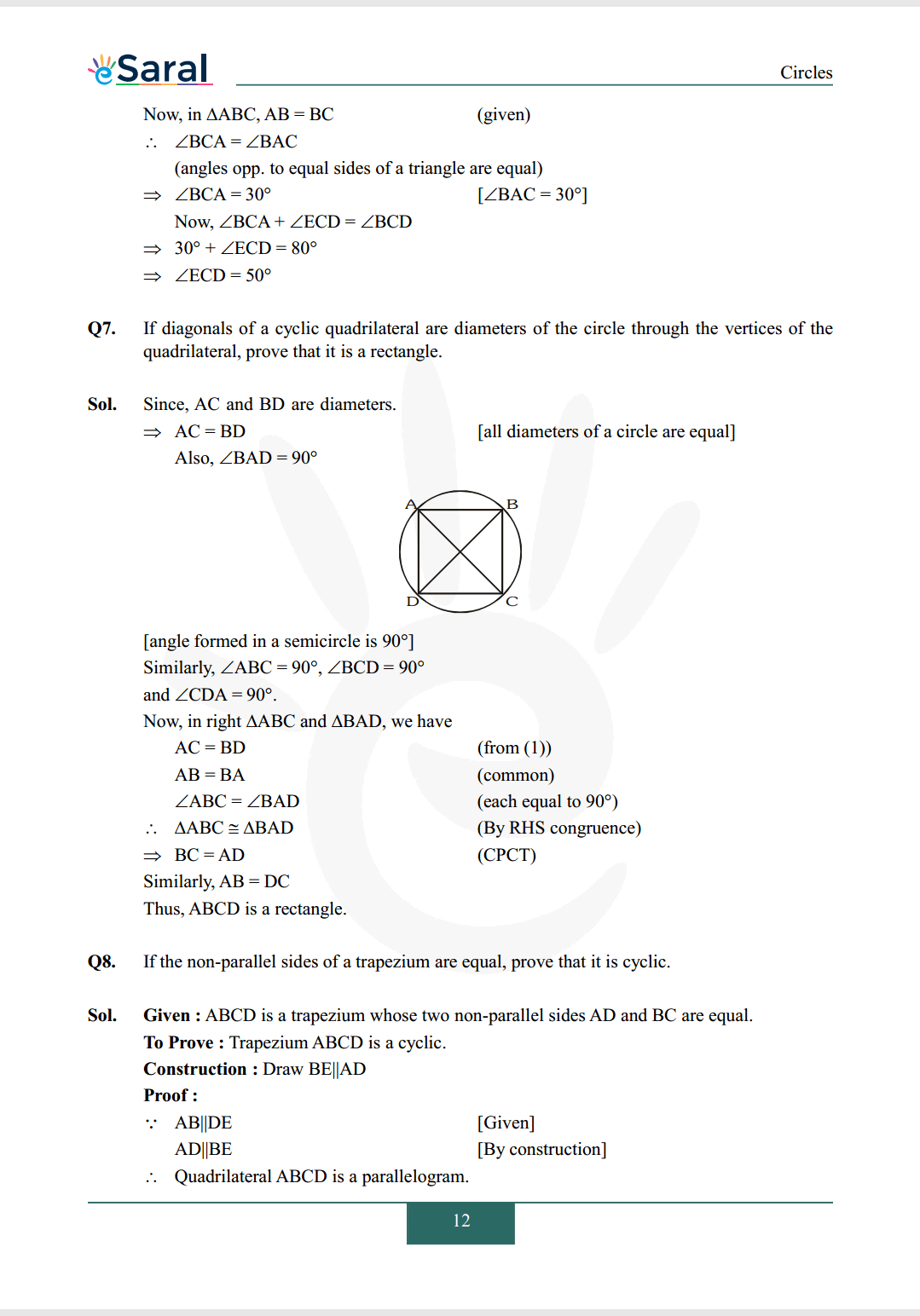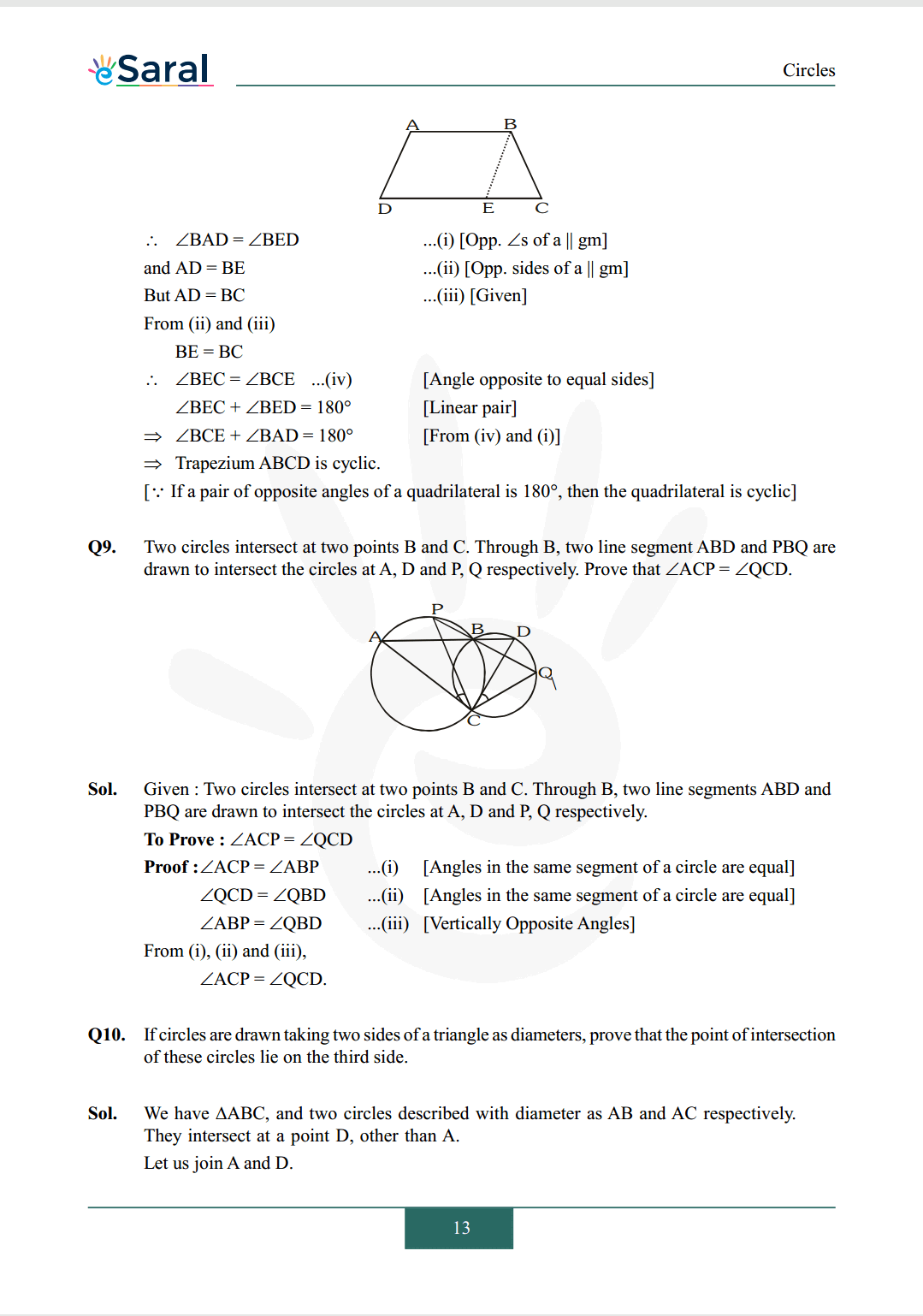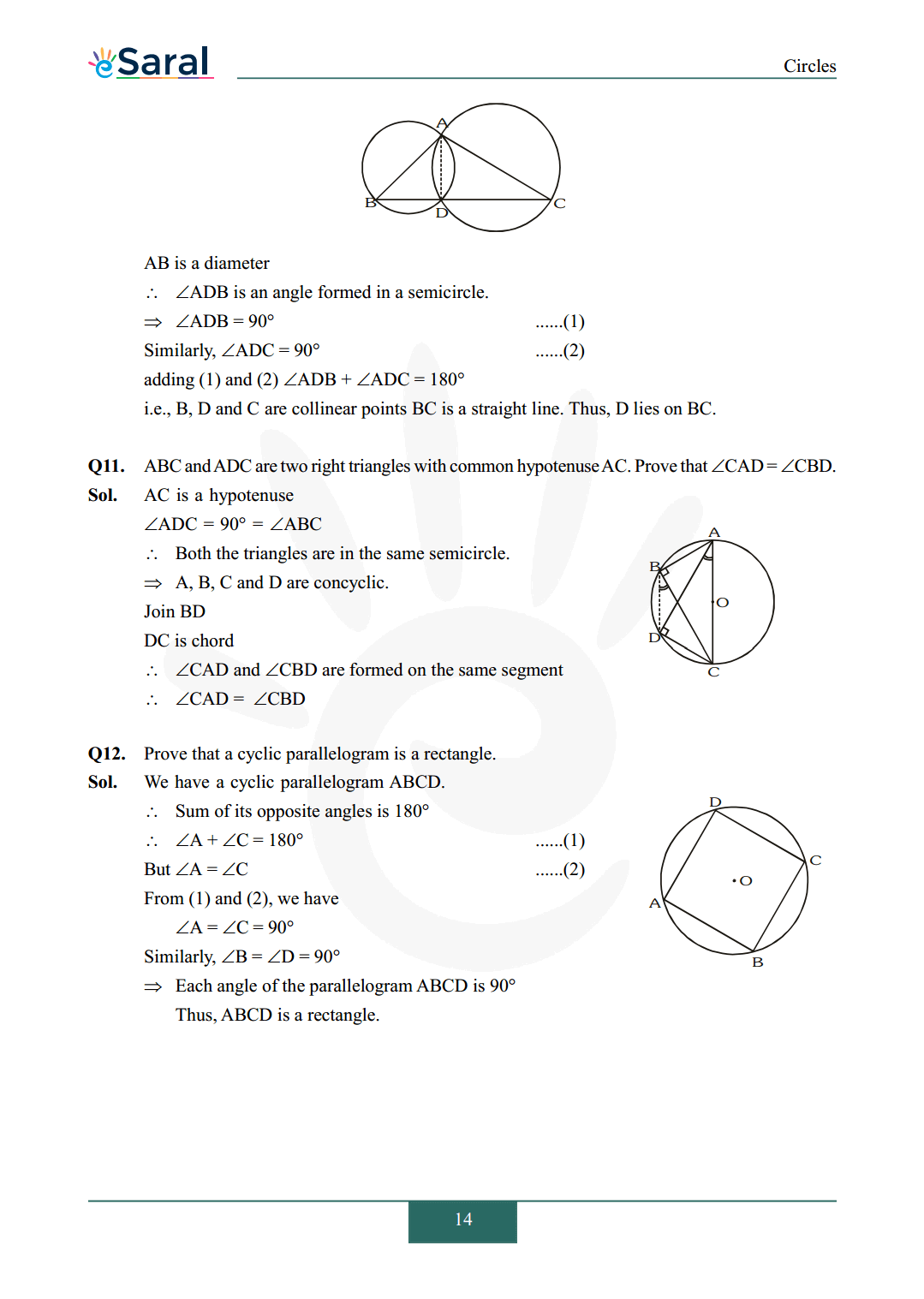#### All Questions of Chapter 10 Exercise 10.5

Once you complete the chapter 10 then you can revise Ex. 10.5 by solving following questions

Q1. In Fig. A, B and C are three points on a circle with centre $\mathrm{O}$ such that $\angle \mathrm{BOC}=30^{\circ}$ and $\angle \mathrm{AOB}=60^{\circ} .$ If $\mathrm{D}$ is a point on the circle other than the arc $\angle \mathrm{ABC}$, find $\angle \mathrm{ADC}$.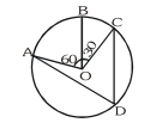Q2. A chord of a circle is equal to the radius of the circle. Find the angle subtended by the chord at a point on the minor arc and also at a point on the major arc.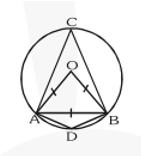Q3. In figure, $\angle \mathrm{PQR}=100^{\circ}$, where $\mathrm{P}, \mathrm{Q}$ and $\mathrm{R}$ are points on a circle with centre $\mathrm{O}$. Find $\angle \mathrm{OPR}$.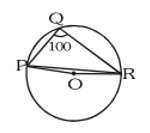Q4. In fig. $\angle \mathrm{ABC}=69^{\circ}, \angle \mathrm{ACB}=31^{\circ}$, find $\angle \mathrm{BDC}$.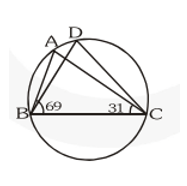Q5. In figure, $\mathrm{A}, \mathrm{B}, \mathrm{C}$ and $\mathrm{D}$ are four points on a circle. AC and BD intersect at a point $\mathrm{E}$ such that $\angle \mathrm{BEC}=130^{\circ}$ and $\angle \mathrm{ECD}=20^{\circ} .$ Find $\angle \mathrm{BAC}$.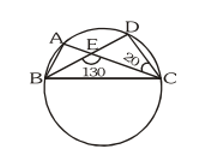Q6. $\quad \mathrm{ABCD}$ is a cyclic quadrilateral whose diagonals intersect at a point $\mathrm{E}$. If $\angle \mathrm{DBC}=70^{\circ}$, $\angle \mathrm{BAC}=$ is $30^{\circ}$, find $\angle \mathrm{BCD}$. Further, if $\mathrm{AB}=\mathrm{BC}$, find $\angle \mathrm{ECD}$.

Q7. If diagonals of a cyclic quadrilateral are diameters of the circle through the vertices of the quadrilateral, prove that it is a rectangle.

Q8. If the non-parallel sides of a trapezium are equal, prove that it is cyclic.

Q9. Two circles intersect at two points $\mathrm{B}$ and $\mathrm{C}$. Through $\mathrm{B}$, two line segment $\mathrm{ABD}$ and $\mathrm{PBQ}$ are drawn to intersect the circles at $\mathrm{A}, \mathrm{D}$ and $\mathrm{P}, \mathrm{Q}$ respectively. Prove that $\angle \mathrm{ACP}=\angle \mathrm{QCD}$.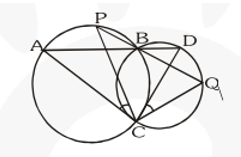Q10. If circles are drawn taking two sides of a triangle as diameters, prove that the point of intersection of these circles lie on the third side.

Q11. $\mathrm{ABC}$ and $\mathrm{ADC}$ are two right triangles with common hypotenuse $\mathrm{AC}$. Prove that $\angle \mathrm{CAD}=\angle \mathrm{CBD}$.

Q12. Prove that a cyclic parallelogram is a rectangle.